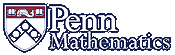Math 425: Partial Differential Equations### SOME REFERENCES

#### Our Text:

• Haberman, Richard, Applied Partial Differential Equations with Fourier Series and Boundary Value Problems, 5th Edition 2012, Pearson/Prentice Hall, Publishers

#### Alternates

• J. David Logan, Applied Mathematics, Wiley-Interscience, 1987. A reasonable alternative text for this course.

• Tikhonov and Samarskii, Equations of Mathematical Physics, translated from the Russian, Pergamon Press, 1963. Reprinted by Dover Publications, 1990. [Includes some background on applications].

• Weinberger, H. L. A First Course in Partial Differential Equations: with Complex Variables and Transform Methods, Dover paperback (paperback reprinted from the 1965 edition); ISBN-13: 978-0486686400

• Murray, J. D., Mathematical Biology I: an Introduction Third Edition, Springer (2001)
________, Mathematical Biology II: Spacial models and Biomedical Applications Beautiful Examples.

• Strauss, Walter A., Partial differential equations: An Introduction, 2nd Edition, John Wiley (2007). This is the standard text for Math 425
• Carrier, G. F. and C. E. Pearson, Partial Differential Equations, Academic Press, 1976; Second edition, 1988. [Less theoretical than Strauss, aimed at people trying to solve practical problems.]

• DiBenedetto, E., Partial Differential Equations, Birkhauser, 1995.

• Evans, Lawrence C., Partial Differential Equations, in the series Graduate studies in mathematics, v. 19, American Math. Society, Second Edition, 2010. Standard text for a graduate math course.

• Friedrichs, K.O., Lecture Notes on Wave Propagatgation Classical 1951 Lecture Notes (scanned PDF, approx 12MB for each part)).
Part 1,   Part 1I,   Part 1II,   Part 1V.

• John, F., Partial Differential Equations, 4th edition, Springer Applied Mathematics Series, 1982.

• McOwen, R., Partial Differential Equations: Methods and Applications, Prentice-Hall, 1996

• Renardy, Michael and Rogers, Robert C., An Introduction to Partial Differential Equations, New York: Springer-Verlag, 1993, Texts in Applied Mathematics; 13.